# NCERT Exemplar Class 11 Chemistry Solutions for Chapter 7 - Equilibrium

## NCERT Exemplar Solutions Class 11 Chemistry Chapter 7 – Free PDF Download

NCERT Exemplar Solutions for Chemistry Class 11 Chapter 7 Equilibrium help you understand the basic concepts of equilibrium in an interactive manner. Equilibrium is a fundamental topic that is necessary for students to understand in order to score well in the exams and to understand advanced topics in the future. The NCERT Exemplar Class 11 Chemistry PDF presents answers to questions in the NCERT Exemplar book, along with Class 11 important questions, Chemical Equilibrium IIT JEE questions and problems with solutions which will guide you in preparing Chemical Equilibrium Class 11 notes for your exam. Once students get familiar with the exemplar, they will understand the important topics to study and prepare to tackle the questions that can be asked in the exams.

In Chapter 7, students will learn about the topic of equilibrium. Generally, the term equilibrium in Chemistry is described as a state of a reaction where both forward and reverse reactions are balanced, and there are no new changes in the overall process. However, in Class 11, students will be exploring the topic in detail, and they will have to study numerous laws, characteristics of equilibria, ionisation, pH scale and more. Studying these topics can get quite difficult. In order to guide students in mastering all chapter concepts, we are offering free NCERT Exemplar for Class 11 Chemistry Chapter 7 – Equilibrium here. The NCERT Exemplar has been designed carefully by the subject experts to help students learn about all the topics in an efficient way. The solutions also consist of clear and concise answers that will help students complete their revisions in time.

### Download the PDF of the NCERT Exemplar for Class 11 Chemistry Chapter 7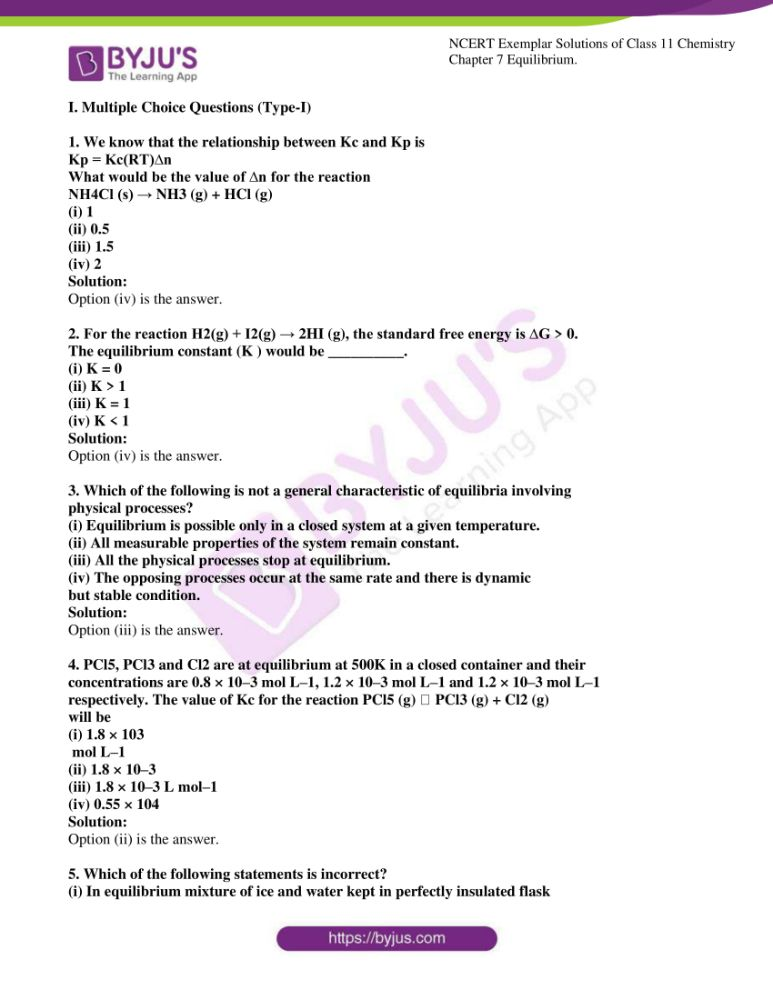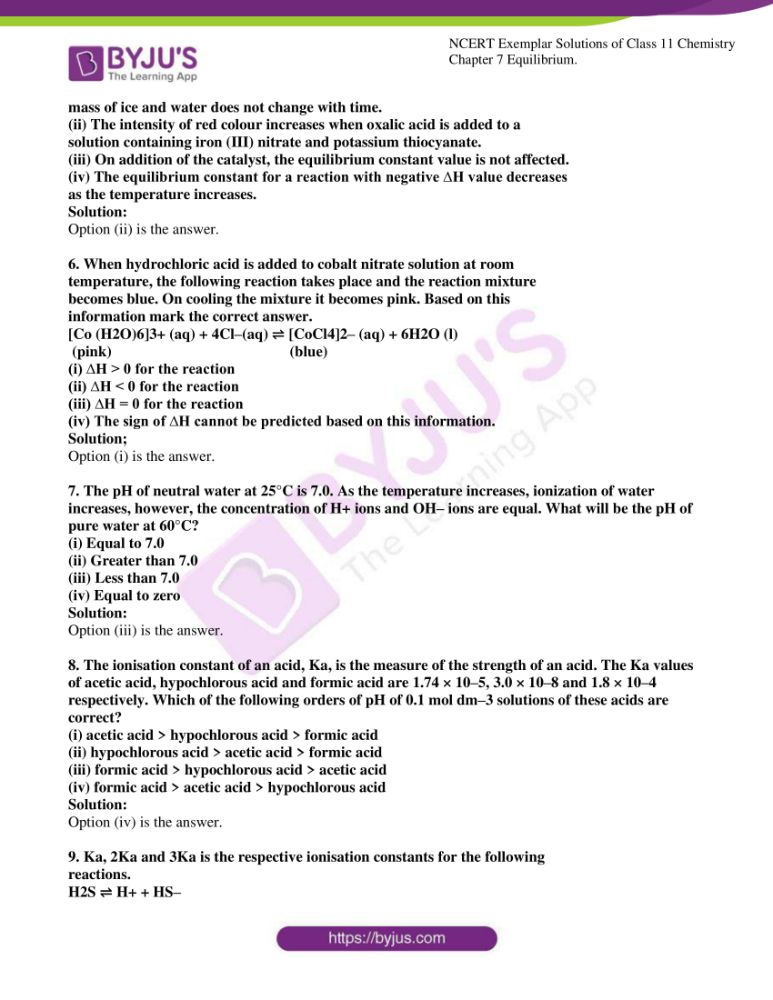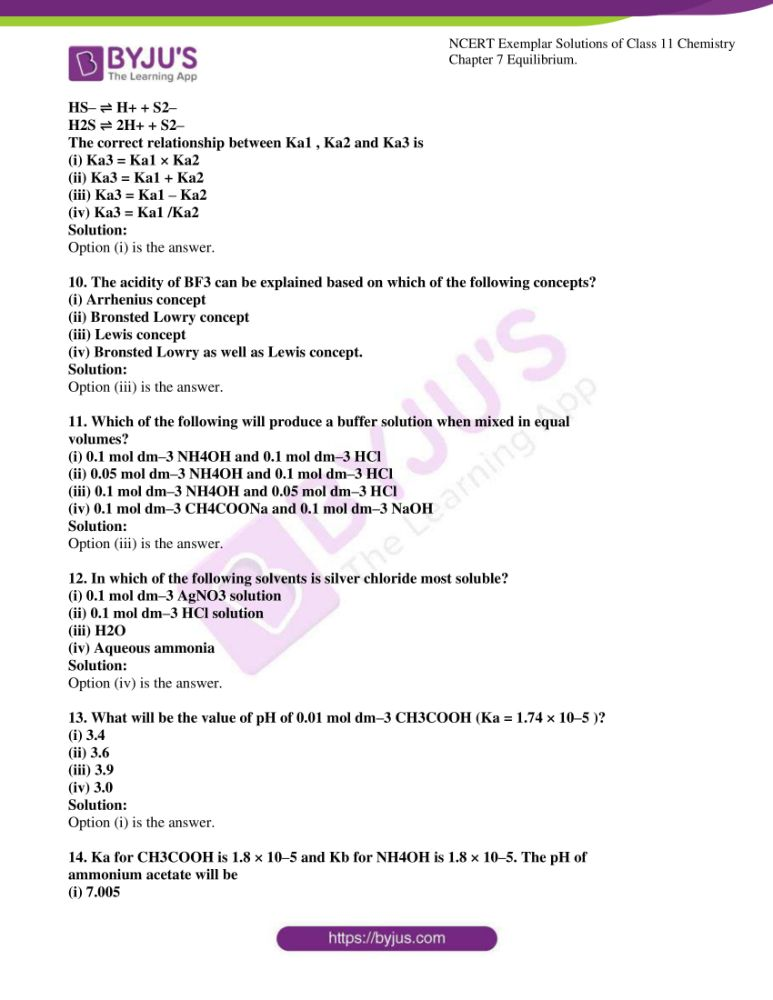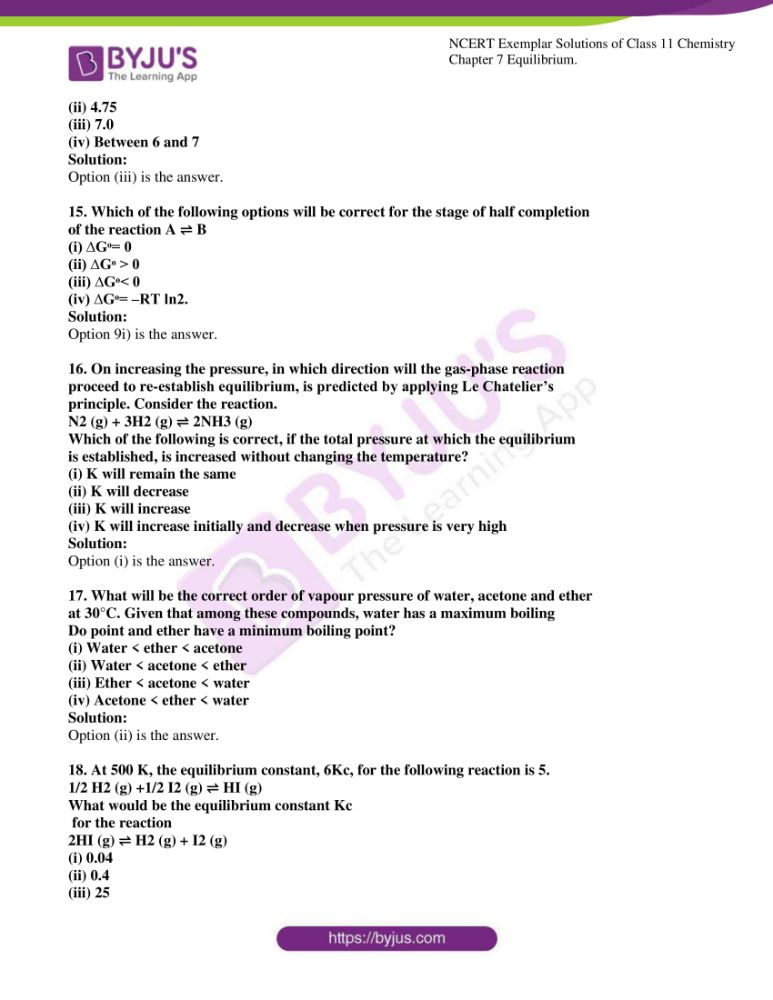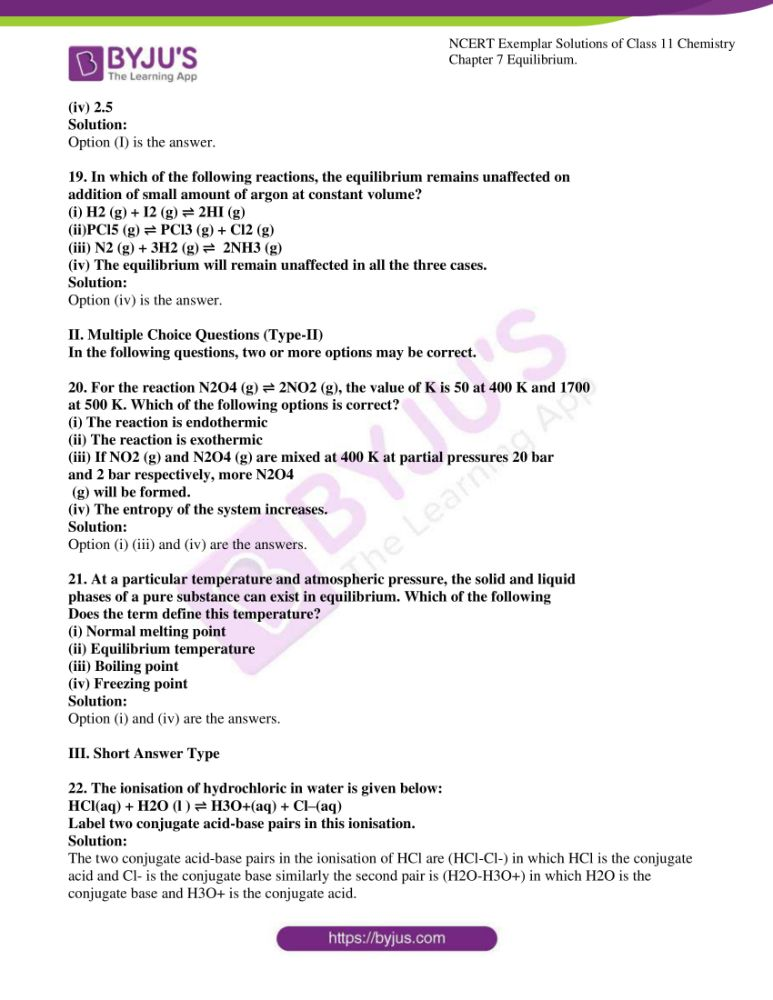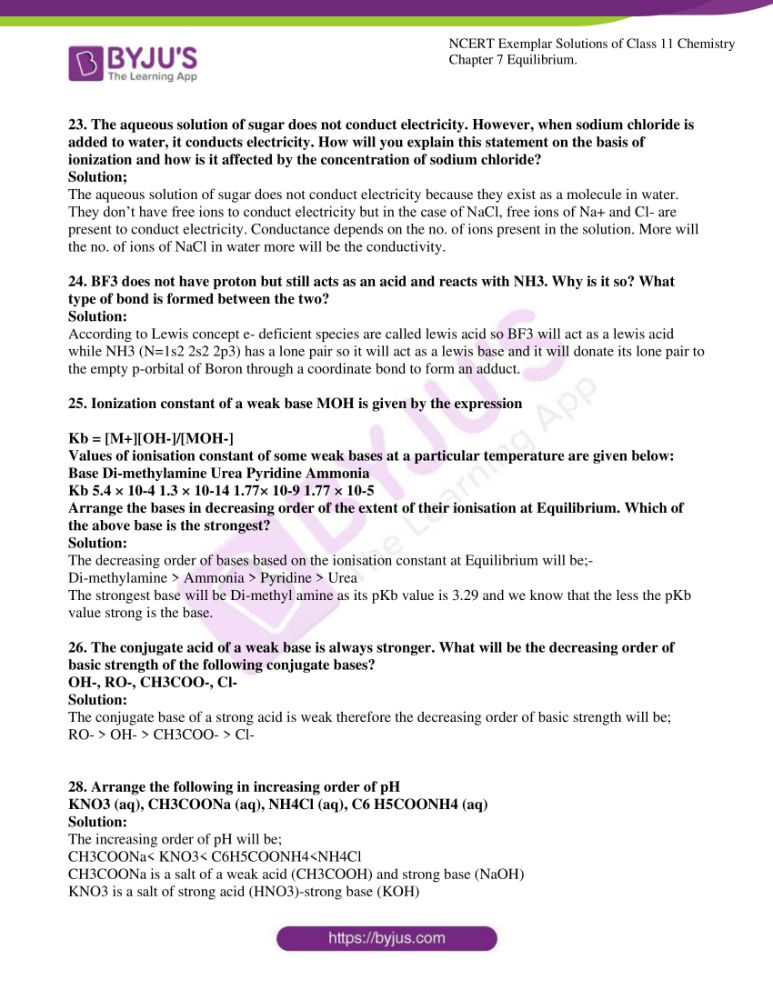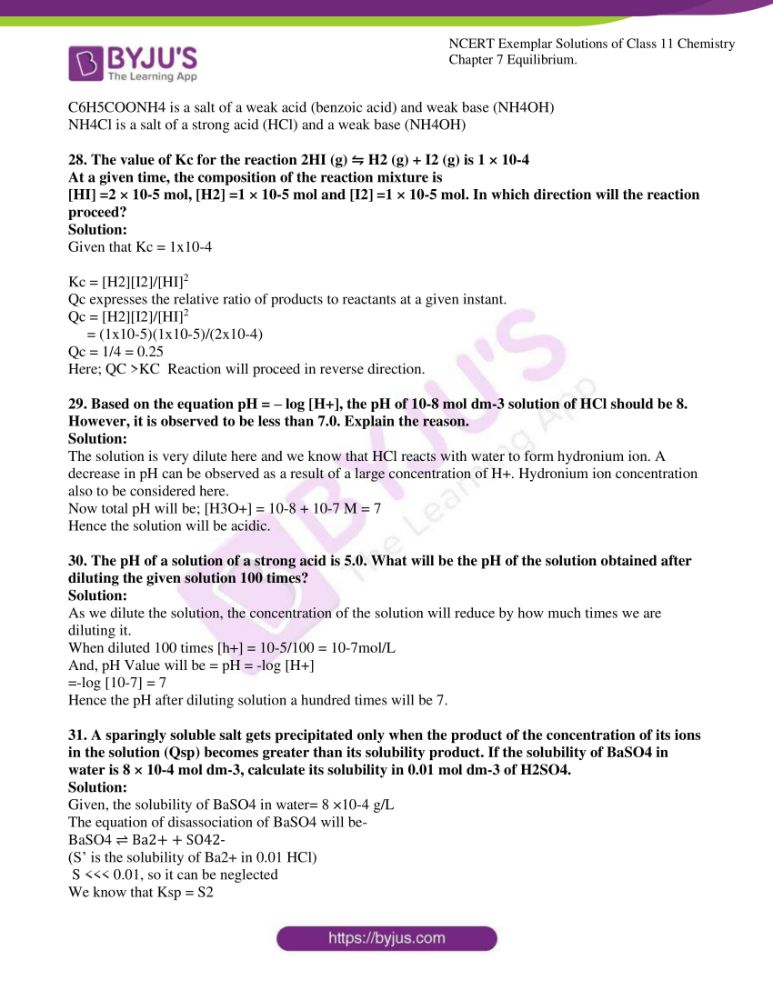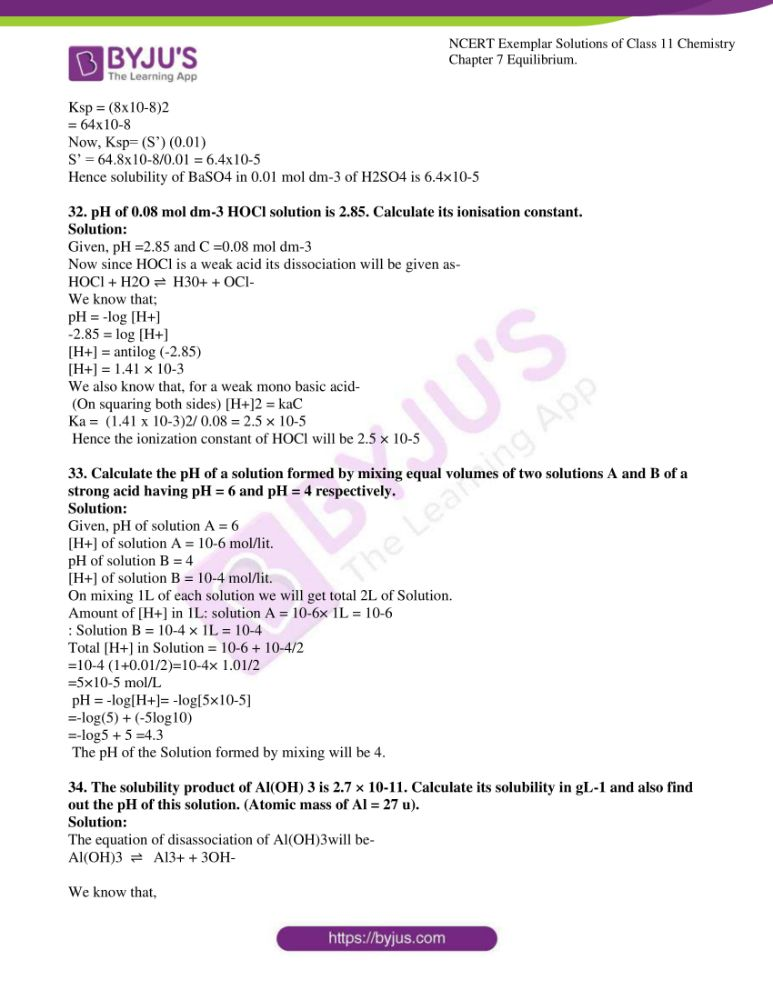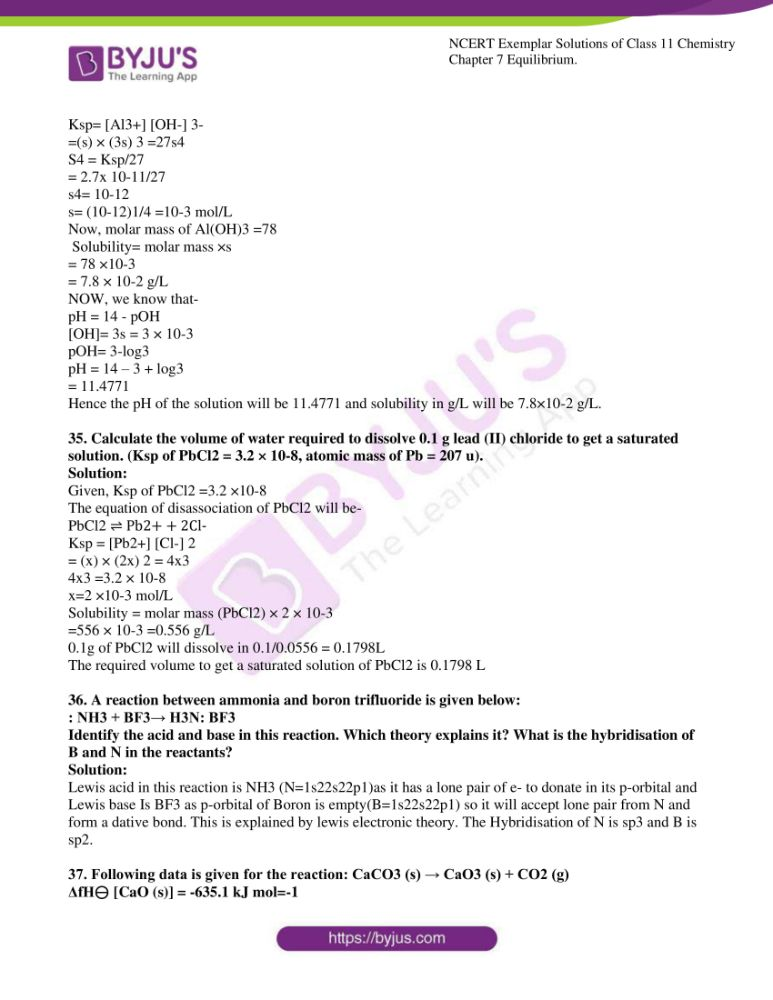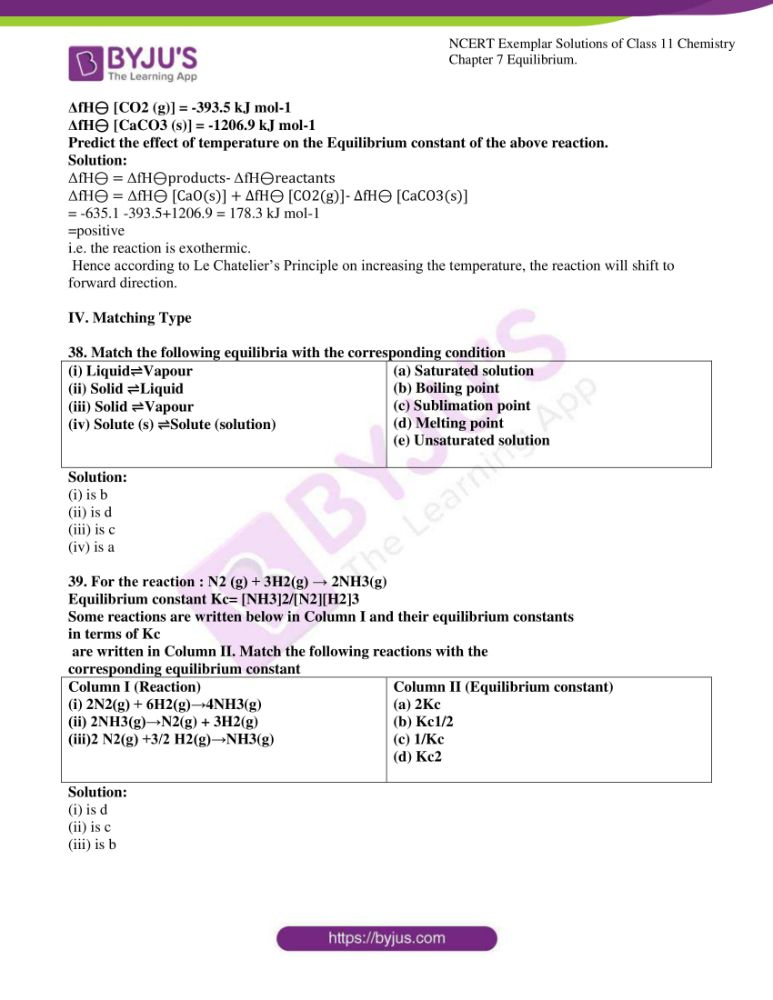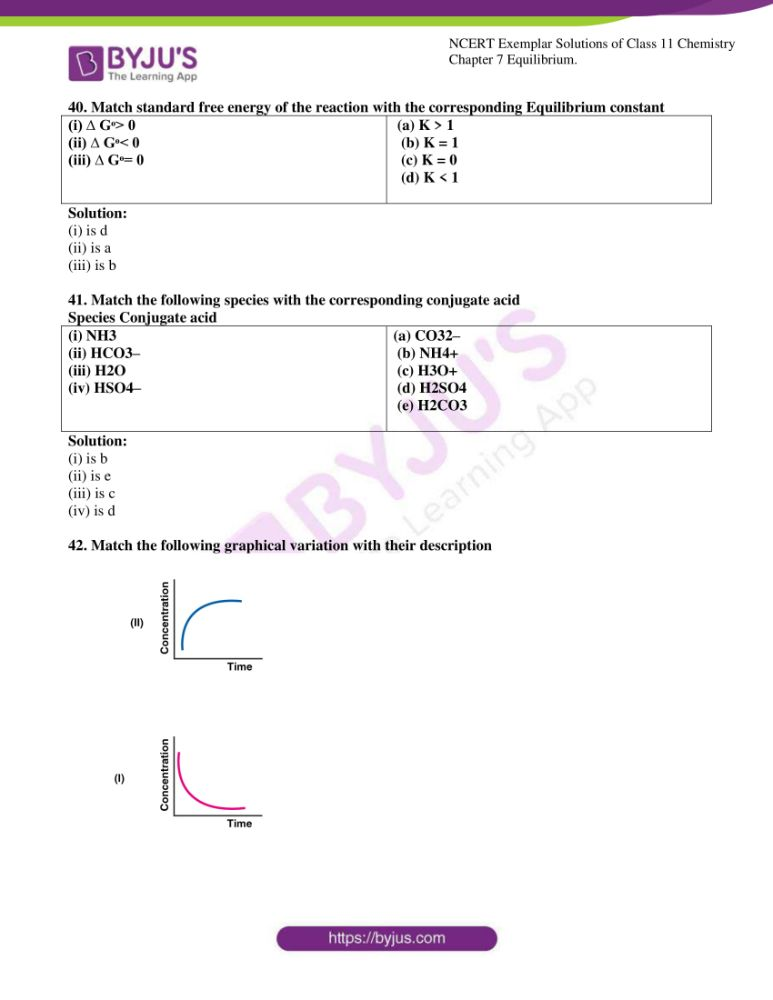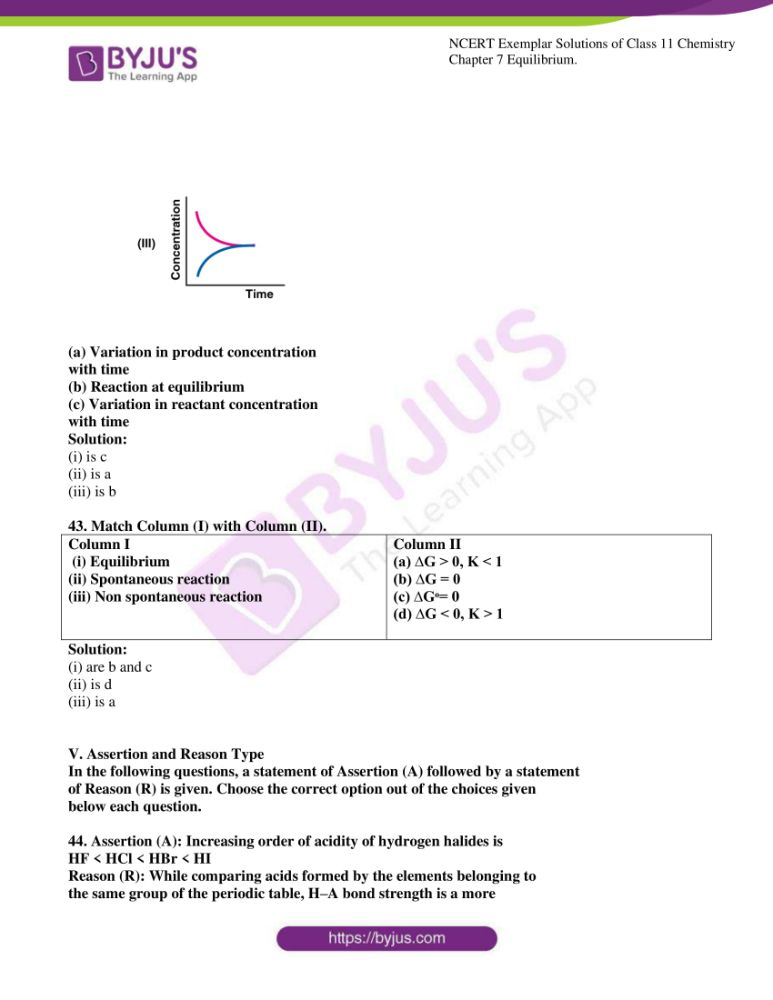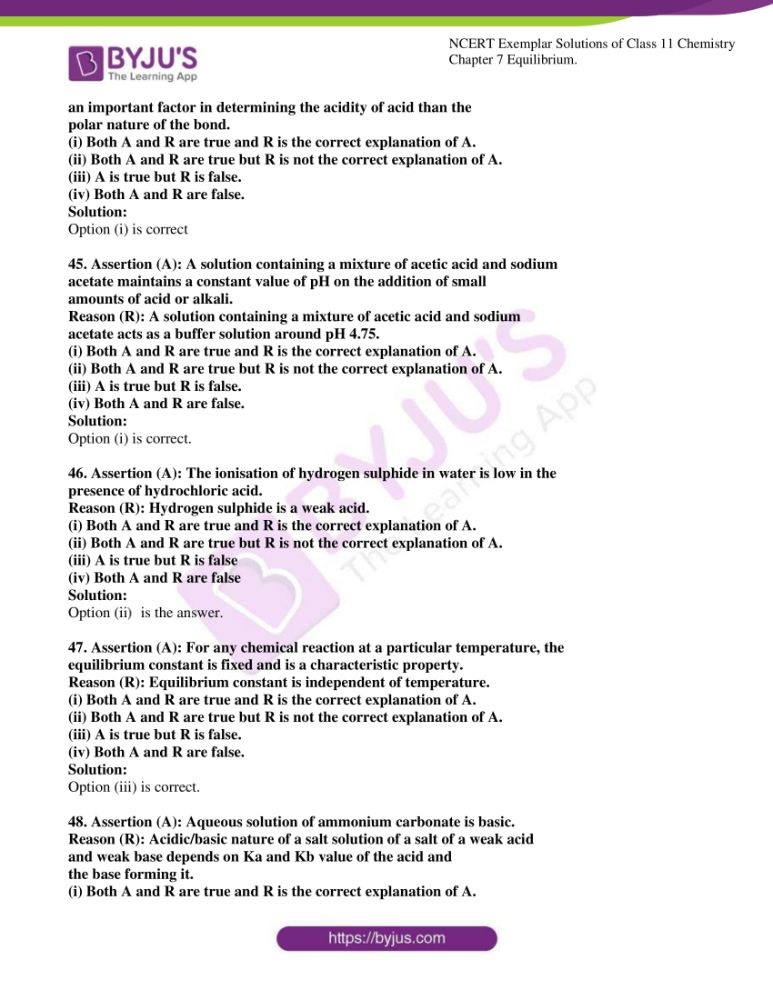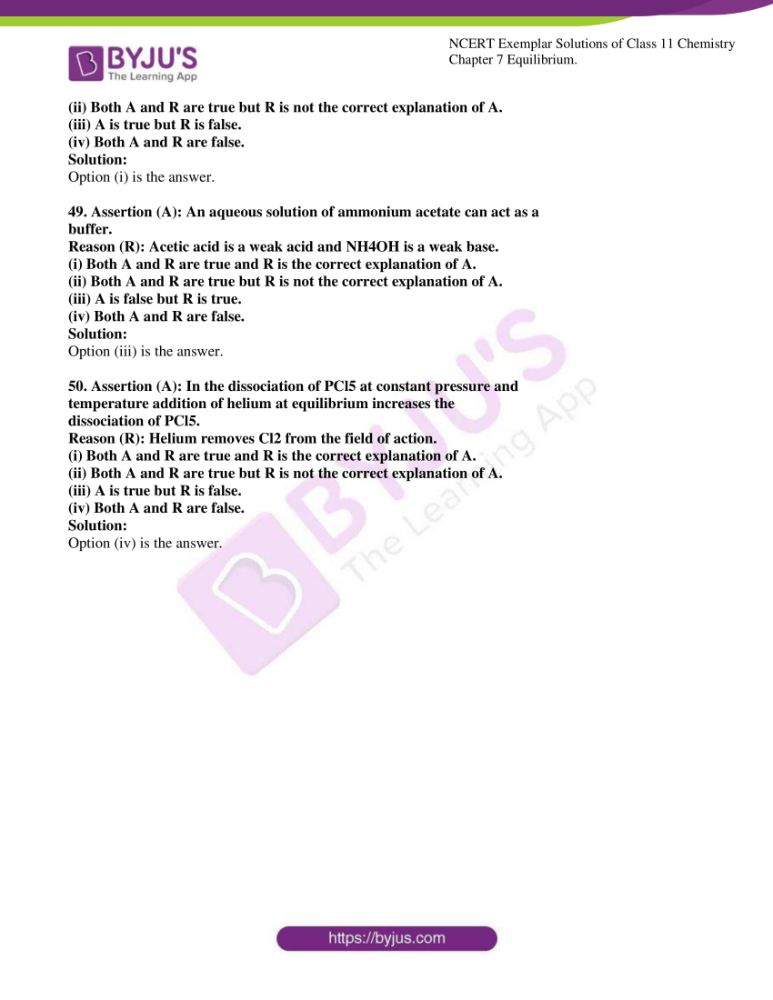### Access Answers to the NCERT Exemplar for Class 11 Chemistry Chapter 7

I. Multiple Choice Questions (Type-I)

1. We know that the relationship between Kc and Kp is

Kp = Kc(RT)∆n

What would be the value of ∆n for the reaction

NH4Cl (s) → NH3 (g) + HCl (g)

(i) 1

(ii) 0.5

(iii) 1.5

(iv) 2

Solution:

2. For the reaction H2(g) + I2(g) → 2HI (g), the standard free energy is ∆G > 0.

The equilibrium constant (K ) would be __________.

(i) K = 0

(ii) K > 1

(iii) K = 1

(iv) K < 1

Solution:

3. Which of the following is not a general characteristic of equilibria involving

physical processes?

(i) Equilibrium is possible only in a closed system at a given temperature.

(ii) All measurable properties of the system remain constant.

(iii) All the physical processes stop at equilibrium.

(iv) The opposing processes occur at the same rate and there is dynamic

but stable condition.

Solution:

4. PCl5, PCl3 and Cl2 are at equilibrium at 500K in a closed container and their

concentrations are 0.8 × 10–3 mol L–1, 1.2 × 10–3 mol L–1 and 1.2 × 10–3 mol L–1

respectively. The value of Kc for the reaction PCl5 (g)  PCl3 (g) + Cl2 (g)

will be

(i) 1.8 × 103

mol L–1

(ii) 1.8 × 10–3

(iii) 1.8 × 10–3 L mol–1

(iv) 0.55 × 104

Solution:

5. Which of the following statements is incorrect?

(i) In equilibrium mixture of ice and water kept in perfectly insulated flask

mass of ice and water does not change with time.

(ii) The intensity of red colour increases when oxalic acid is added to a

solution containing iron (III) nitrate and potassium thiocyanate.

(iii) On addition of the catalyst, the equilibrium constant value is not affected.

(iv) The equilibrium constant for a reaction with negative ∆H value decreases

as the temperature increases.

Solution:

6. When hydrochloric acid is added to cobalt nitrate solution at room

temperature, the following reaction takes place and the reaction mixture

becomes blue. On cooling the mixture it becomes pink. Based on this

[Co (H2O)6]3+ (aq) + 4Cl–(aq) ⇌ [CoCl4]2– (aq) + 6H2O (l)

(pink) (blue)

(i) ∆H > 0 for the reaction

(ii) ∆H < 0 for the reaction

(iii) ∆H = 0 for the reaction

(iv) The sign of ∆H cannot be predicted based on this information.

Solution;

7. The pH of neutral water at 25°C is 7.0. As the temperature increases, ionization of water increases, however, the concentration of H+ ions and OH– ions are equal. What will be the pH of pure water at 60°C?

(i) Equal to 7.0

(ii) Greater than 7.0

(iii) Less than 7.0

(iv) Equal to zero

Solution:

8. The ionisation constant of an acid, Ka, is the measure of the strength of an acid. The Ka values of acetic acid, hypochlorous acid and formic acid are 1.74 × 10–5, 3.0 × 10–8 and 1.8 × 10–4 respectively. Which of the following orders of pH of 0.1 mol dm–3 solutions of these acids are correct?

(i) acetic acid > hypochlorous acid > formic acid

(ii) hypochlorous acid > acetic acid > formic acid

(iii) formic acid > hypochlorous acid > acetic acid

(iv) formic acid > acetic acid > hypochlorous acid

Solution:

9. Ka, 2Ka and 3Ka is the respective ionisation constants for the following

reactions.

H2S ⇌ H+ + HS–

HS– ⇌ H+ + S2–

H2S ⇌ 2H+ + S2–

The correct relationship between Ka1 , Ka2 and Ka3 is

(i) Ka3 = Ka1 × Ka2

(ii) Ka3 = Ka1 + Ka2

(iii) Ka3 = Ka1 – Ka2

(iv) Ka3 = Ka1 /Ka2

Solution:

10. The acidity of BF3 can be explained based on which of the following concepts?

(i) Arrhenius concept

(ii) Bronsted Lowry concept

(iii) Lewis concept

(iv) Bronsted Lowry as well as Lewis concept.

Solution:

11. Which of the following will produce a buffer solution when mixed in equal

volumes?

(i) 0.1 mol dm–3 NH4OH and 0.1 mol dm–3 HCl

(ii) 0.05 mol dm–3 NH4OH and 0.1 mol dm–3 HCl

(iii) 0.1 mol dm–3 NH4OH and 0.05 mol dm–3 HCl

(iv) 0.1 mol dm–3 CH4COONa and 0.1 mol dm–3 NaOHSolution:

12. In which of the following solvents is silver chloride most soluble?

(i) 0.1 mol dm–3 AgNO3 solution

(ii) 0.1 mol dm–3 HCl solution

(iii) H2O

(iv) Aqueous ammoniaSolution:

13. What will be the value of pH of 0.01 mol dm–3 CH3COOH (Ka = 1.74 × 10–5 )?

(i) 3.4

(ii) 3.6

(iii) 3.9

(iv) 3.0

Solution:

14. Ka for CH3COOH is 1.8 × 10–5 and Kb for NH4OH is 1.8 × 10–5. The pH of

ammonium acetate will be

(i) 7.005

(ii) 4.75

(iii) 7.0

(iv) Between 6 and 7

Solution:

15. Which of the following options will be correct for the stage of half completion

of the reaction A ⇌ B

(i) ∆Gᶱ= 0

(ii) ∆Gᶱ > 0

(iii) ∆Gᶱ< 0

(iv) ∆Gᶱ= –RT ln2.

Solution:

16. On increasing the pressure, in which direction will the gas-phase reaction

proceed to re-establish equilibrium, is predicted by applying Le Chatelier’s

principle. Consider the reaction.

N2 (g) + 3H2 (g) ⇌ 2NH3 (g)

Which of the following is correct, if the total pressure at which the equilibrium

is established, is increased without changing the temperature?

(i) K will remain the same

(ii) K will decrease

(iii) K will increase

(iv) K will increase initially and decrease when pressure is very highSolution:

17. What will be the correct order of vapour pressure of water, acetone and ether

at 30°C. Given that among these compounds, water has a maximum boiling

Do point and ether have a minimum boiling point?

(i) Water < ether < acetone

(ii) Water < acetone < ether

(iii) Ether < acetone < water

(iv) Acetone < ether < water

Solution:

18. At 500 K, the equilibrium constant, 6Kc, for the following reaction is 5.

1/2 H2 (g) +1/2 I2 (g) ⇌ HI (g)

What would be the equilibrium constant Kc

for the reaction

2HI (g) ⇌ H2 (g) + I2 (g)

(i) 0.04

(ii) 0.4

(iii) 25

(iv) 2.5

Solution:

19. In which of the following reactions, the equilibrium remains unaffected on

addition of small amount of argon at constant volume?

(i) H2 (g) + I2 (g) ⇌ 2HI (g)

(ii)PCl5 (g) ⇌ PCl3 (g) + Cl2 (g)

(iii) N2 (g) + 3H2 (g) ⇌ 2NH3 (g)

(iv) The equilibrium will remain unaffected in all the three cases.

Solution:

II. Multiple Choice Questions (Type-II)

In the following questions, two or more options may be correct.

20. For the reaction N2O4 (g) ⇌ 2NO2 (g), the value of K is 50 at 400 K and 1700

at 500 K. Which of the following options is correct?

(i) The reaction is endothermic

(ii) The reaction is exothermic

(iii) If NO2 (g) and N2O4 (g) are mixed at 400 K at partial pressures 20 bar

and 2 bar respectively, more N2O4

(g) will be formed.

(iv) The entropy of the system increases.

Solution:

Option (i) (iii) and (iv) are the answers.

21. At a particular temperature and atmospheric pressure, the solid and liquid

phases of a pure substance can exist in equilibrium. Which of the following

Does the term define this temperature?

(i) Normal melting point

(ii) Equilibrium temperature

(iii) Boiling point

(iv) Freezing point

Solution:

Option (i) and (iv) are the answers.

22. The ionisation of hydrochloric in water is given below:

HCl(aq) + H2O (l ) ⇌ H3O+(aq) + Cl–(aq)

Label two conjugate acid-base pairs in this ionisation.

Solution:

The two conjugate acid-base pairs in the ionisation of HCl are (HCl-Cl-) in which HCl is the conjugate acid and Cl- is the conjugate base similarly the second pair is (H2O-H3O+) in which H2O is the conjugate base and H3O+ is the conjugate acid.

23. The aqueous solution of sugar does not conduct electricity. However, when sodium chloride is added to water, it conducts electricity. How will you explain this statement on the basis of ionization and how is it affected by the concentration of sodium chloride?

Solution;

The aqueous solution of sugar does not conduct electricity because they exist as a molecule in water. They don’t have free ions to conduct electricity but in the case of NaCl, free ions of Na+ and Cl- are present to conduct electricity. Conductance depends on the no. of ions present in the solution. More will the no. of ions of NaCl in water more will be the conductivity.

24. BF3 does not have proton but still acts as an acid and reacts with NH3. Why is it so? What type of bond is formed between the two?

Solution:

According to Lewis concept e- deficient species are called lewis acid so BF3 will act as a lewis acid while NH3 (N=1s2 2s2 2p3) has a lone pair so it will act as a lewis base and it will donate its lone pair to the empty p-orbital of Boron through a coordinate bond to form an adduct.

25. Ionization constant of a weak base MOH is given by the expression

Kb = [M+][OH-]/[MOH-]

Values of ionisation constant of some weak bases at a particular temperature are given below:

Base Di-methylamine Urea Pyridine Ammonia

Kb 5.4 × 10-4 1.3 × 10-14 1.77× 10-9 1.77 × 10-5

Arrange the bases in decreasing order of the extent of their ionisation at Equilibrium. Which of the above base is the strongest?

Solution:

The decreasing order of bases based on the ionisation constant at Equilibrium will be;-

Di-methylamine > Ammonia > Pyridine > Urea

The strongest base will be Di-methyl amine as its pKb value is 3.29 and we know that the less the pKb value strong is the base.

26. The conjugate acid of a weak base is always stronger. What will be the decreasing order of basic strength of the following conjugate bases?

OH-, RO-, CH3COO-, Cl-

Solution:

The conjugate base of a strong acid is weak therefore the decreasing order of basic strength will be;

RO- > OH- > CH3COO- > Cl-

28. Arrange the following in increasing order of pH

KNO3 (aq), CH3COONa (aq), NH4Cl (aq), C6 H5COONH4 (aq)

Solution:

The increasing order of pH will be;

CH3COONa< KNO3< C6H5COONH4<NH4Cl

CH3COONa is a salt of a weak acid (CH3COOH) and strong base (NaOH)

KNO3 is a salt of strong acid (HNO3)-strong base (KOH)

C6H5COONH4 is a salt of a weak acid (benzoic acid) and weak base (NH4OH)

NH4Cl is a salt of a strong acid (HCl) and a weak base (NH4OH)

28. The value of Kc for the reaction 2HI (g) ⇋ H2 (g) + I2 (g) is 1 × 10-4

At a given time, the composition of the reaction mixture is

[HI] =2 × 10-5 mol, [H2] =1 × 10-5 mol and [I2] =1 × 10-5 mol. In which direction will the reaction proceed?

Solution:

Given that Kc = 1×10-4

Kc = [H2][I2]/[HI]2

Qc expresses the relative ratio of products to reactants at a given instant.

Qc = [H2][I2]/[HI]2

= (1×10-5)(1×10-5)/(2×10-4)

Qc = 1/4 = 0.25

Here; QC >KC Reaction will proceed in reverse direction.

29. Based on the equation pH = – log [H+], the pH of 10-8 mol dm-3 solution of HCl should be 8. However, it is observed to be less than 7.0. Explain the reason.

Solution:

The solution is very dilute here and we know that HCl reacts with water to form hydronium ion. A decrease in pH can be observed as a result of a large concentration of H+. Hydronium ion concentration also to be considered here.

Now total pH will be; [H3O+] = 10-8 + 10-7 M = 7

Hence the solution will be acidic.

30. The pH of a solution of a strong acid is 5.0. What will be the pH of the solution obtained after diluting the given solution 100 times?

Solution:

As we dilute the solution, the concentration of the solution will reduce by how much times we are diluting it.

When diluted 100 times [h+] = 10-5/100 = 10-7mol/L

And, pH Value will be = pH = -log [H+]

=-log [10-7] = 7

Hence the pH after diluting solution a hundred times will be 7.

31. A sparingly soluble salt gets precipitated only when the product of the concentration of its ions in the solution (Qsp) becomes greater than its solubility product. If the solubility of BaSO4 in water is 8 × 10-4 mol dm-3, calculate its solubility in 0.01 mol dm-3 of H2SO4.

Solution:

Given, the solubility of BaSO4 in water= 8 ×10-4 g/L

The equation of disassociation of BaSO4 will be-

BaSO4 ⇌ Ba2+ + SO42-

(S’ is the solubility of Ba2+ in 0.01 HCl)

S <<< 0.01, so it can be neglected

We know that Ksp = S2

Ksp = (8×10-8)2

= 64×10-8

Now, Ksp= (S’) (0.01)

S’ = 64.8×10-8/0.01 = 6.4×10-5

Hence solubility of BaSO4 in 0.01 mol dm-3 of H2SO4 is 6.4×10-5

32. pH of 0.08 mol dm-3 HOCl solution is 2.85. Calculate its ionisation constant.

Solution:

Given, pH =2.85 and C =0.08 mol dm-3

Now since HOCl is a weak acid its dissociation will be given as-

HOCl + H2O ⇌ H30+ + OCl-

We know that;

pH = -log [H+]

-2.85 = log [H+] [H+] = antilog (-2.85)

[H+] = 1.41 × 10-3

We also know that, for a weak mono basic acid-

(On squaring both sides) [H+]2 = kaC

Ka = (1.41 x 10-3)2/ 0.08 = 2.5 × 10-5

Hence the ionization constant of HOCl will be 2.5 × 10-5

33. Calculate the pH of a solution formed by mixing equal volumes of two solutions A and B of a strong acid having pH = 6 and pH = 4 respectively.

Solution:

Given, pH of solution A = 6

[H+] of solution A = 10-6 mol/lit.

pH of solution B = 4

[H+] of solution B = 10-4 mol/lit.

On mixing 1L of each solution we will get total 2L of Solution.

Amount of [H+] in 1L: solution A = 10-6× 1L = 10-6

: Solution B = 10-4 × 1L = 10-4

Total [H+] in Solution = 10-6 + 10-4/2

=10-4 (1+0.01/2)=10-4× 1.01/2

=5×10-5 mol/L

pH = -log[H+]= -log[5×10-5]

=-log(5) + (-5log10)

=-log5 + 5 =4.3

The pH of the Solution formed by mixing will be 4.

34. The solubility product of Al(OH) 3 is 2.7 × 10-11. Calculate its solubility in gL-1 and also find out the pH of this solution. (Atomic mass of Al = 27 u).

Solution:

The equation of disassociation of Al(OH)3will be-

Al(OH)3 ⇌ Al3+ + 3OH-

We know that,

Ksp= [Al3+] [OH-] 3-

=(s) × (3s) 3 =27s4

S4 = Ksp/27

= 2.7x 10-11/27

s4= 10-12

s= (10-12)1/4 =10-3 mol/L

Now, molar mass of Al(OH)3 =78

Solubility= molar mass ×s

= 78 ×10-3

= 7.8 × 10-2 g/L

NOW, we know that-

pH = 14 – pOH

[OH]= 3s = 3 × 10-3

pOH= 3-log3

pH = 14 – 3 + log3

= 11.4771

Hence the pH of the solution will be 11.4771 and solubility in g/L will be 7.8×10-2 g/L.

35. Calculate the volume of water required to dissolve 0.1 g lead (II) chloride to get a saturated solution. (Ksp of PbCl2 = 3.2 × 10-8, atomic mass of Pb = 207 u).

Solution:

Given, Ksp of PbCl2 =3.2 ×10-8

The equation of disassociation of PbCl2 will be-

PbCl2 ⇌ Pb2+ + 2Cl-

Ksp = [Pb2+] [Cl-] 2

= (x) × (2x) 2 = 4×3

4×3 =3.2 × 10-8

x=2 ×10-3 mol/L

Solubility = molar mass (PbCl2) × 2 × 10-3

=556 × 10-3 =0.556 g/L

0.1g of PbCl2 will dissolve in 0.1/0.0556 = 0.1798L

The required volume to get a saturated solution of PbCl2 is 0.1798 L

36. A reaction between ammonia and boron trifluoride is given below:

: NH3 + BF3→ H3N: BF3

Identify the acid and base in this reaction. Which theory explains it? What is the hybridisation of B and N in the reactants?

Solution:

Lewis acid in this reaction is NH3 (N=1s22s22p1)as it has a lone pair of e- to donate in its p-orbital and Lewis base Is BF3 as p-orbital of Boron is empty(B=1s22s22p1) so it will accept lone pair from N and form a dative bond. This is explained by lewis electronic theory. The Hybridisation of N is sp3 and B is sp2.

37. Following data is given for the reaction: CaCO3 (s) → CaO3 (s) + CO2 (g)

ΔfH⊖ [CaO (s)] = -635.1 kJ mol=-1

ΔfH⊖ [CO2 (g)] = -393.5 kJ mol-1

ΔfH⊖ [CaCO3 (s)] = -1206.9 kJ mol-1

Predict the effect of temperature on the Equilibrium constant of the above reaction.

Solution:

ΔfH⊖ = ΔfH⊖products- ΔfH⊖reactants

ΔfH⊖ = ΔfH⊖ [CaO(s)] + ΔfH⊖ [CO2(g)]- ΔfH⊖ [CaCO3(s)]

= -635.1 -393.5+1206.9 = 178.3 kJ mol-1

=positive

i.e. the reaction is exothermic.

Hence according to Le Chatelier’s Principle on increasing the temperature, the reaction will shift to forward direction.

IV. Matching Type

38. Match the following equilibria with the corresponding condition

 (i) Liquid⇌Vapour (ii) Solid ⇌Liquid (iii) Solid ⇌Vapour (iv) Solute (s) ⇌Solute (solution) (a) Saturated solution (b) Boiling point (c) Sublimation point (d) Melting point (e) Unsaturated solution

Solution:

(i) is b

(ii) is d

(iii) is c

(iv) is a

39. For the reaction : N2 (g) + 3H2(g) → 2NH3(g)

Equilibrium constant Kc= [NH3]2/[N2][H2]3

Some reactions are written below in Column I and their equilibrium constants

in terms of Kc

are written in Column II. Match the following reactions with the

corresponding equilibrium constant

 Column I (Reaction) (i) 2N2(g) + 6H2(g)→4NH3(g) (ii) 2NH3(g)→N2(g) + 3H2(g) (iii)2 N2(g) +3/2 H2(g)→NH3(g) Column II (Equilibrium constant) (a) 2Kc (b) Kc1/2 (c) 1/Kc (d) Kc2

Solution:

(i) is d

(ii) is c

(iii) is b

40. Match standard free energy of the reaction with the corresponding Equilibrium constant

 (i) ∆ Gᶱ> 0 (ii) ∆ Gᶱ< 0 (iii) ∆ Gᶱ= 0 (a) K > 1 (b) K = 1 (c) K = 0 (d) K < 1

Solution:

(i) is d

(ii) is a

(iii) is b

41. Match the following species with the corresponding conjugate acid

Species Conjugate acid

 (i) NH3 (ii) HCO3– (iii) H2O (iv) HSO4– (a) CO32– (b) NH4+ (c) H3O+ (d) H2SO4 (e) H2CO3

Solution:

(i) is b

(ii) is e

(iii) is c

(iv) is d

42. Match the following graphical variation with their description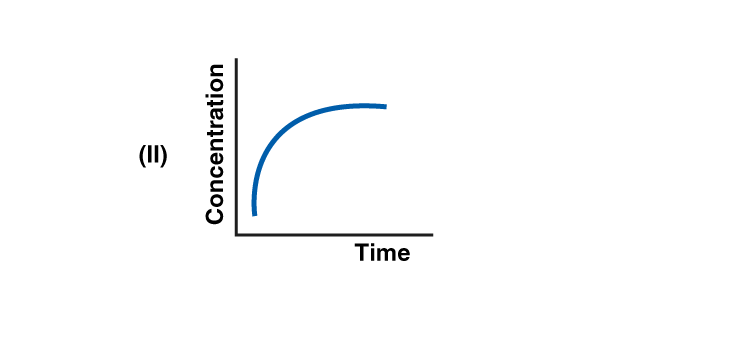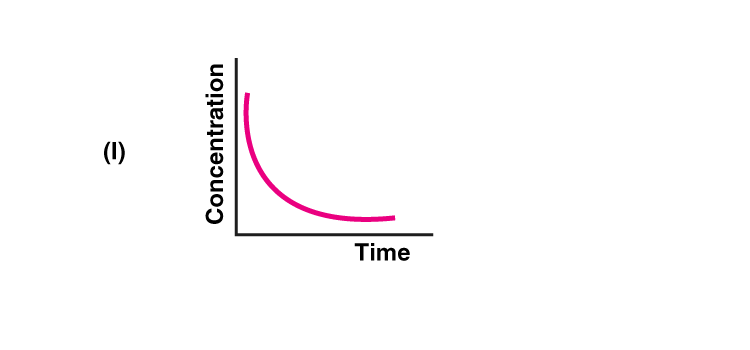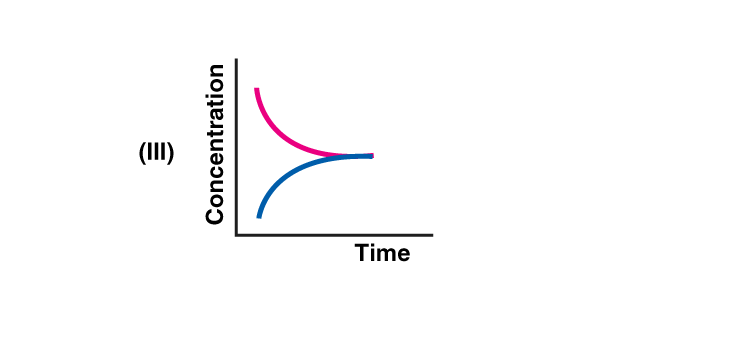(a) Variation in product concentration

with time

(b) Reaction at equilibrium

(c) Variation in reactant concentration

with time

Solution:

(i) is c

(ii) is a

(iii) is b

43. Match Column (I) with Column (II).

 Column I (i) Equilibrium (ii) Spontaneous reaction (iii) Non-spontaneous reaction Column II (a) ∆G > 0, K < 1 (b) ∆G = 0 (c) ∆Gᶱ= 0 (d) ∆G < 0, K > 1

Solution:

(i) are b and c

(ii) is d

(iii) is a

V. Assertion and Reason Type

In the following questions, a statement of Assertion (A) followed by a statement

of Reason (R) is given. Choose the correct option out of the choices given

below each question.

44. Assertion (A): Increasing order of acidity of hydrogen halides is

HF < HCl < HBr < HI

Reason (R): While comparing acids formed by the elements belonging to

the same group of the periodic table, H–A bond strength is a more

an important factor in determining the acidity of acid than the

polar nature of the bond.

(i) Both A and R are true and R is the correct explanation of A.

(ii) Both A and R are true but R is not the correct explanation of A.

(iii) A is true but R is false.

(iv) Both A and R are false.

Solution:

Option (i) is correct

45. Assertion (A): A solution containing a mixture of acetic acid and sodium

acetate maintains a constant value of pH on the addition of small

amounts of acid or alkali.

Reason (R): A solution containing a mixture of acetic acid and sodium

acetate acts as a buffer solution around pH 4.75.

(i) Both A and R are true and R is the correct explanation of A.

(ii) Both A and R are true but R is not the correct explanation of A.

(iii) A is true but R is false.

(iv) Both A and R are false.

Solution:

Option (i) is correct.

46. Assertion (A): The ionisation of hydrogen sulphide in water is low in the

presence of hydrochloric acid.

Reason (R): Hydrogen sulphide is a weak acid.

(i) Both A and R are true and R is the correct explanation of A.

(ii) Both A and R are true but R is not the correct explanation of A.(iii) A is true but R is false

(iv) Both A and R are false

Solution:

47. Assertion (A): For any chemical reaction at a particular temperature,

the equilibrium constant is fixed and is a characteristic property.

Reason (R): Equilibrium constant is independent of temperature.

(i) Both A and R are true and R is the correct explanation of A.

(ii) Both A and R are true but R is not the correct explanation of A.

(iii) A is true but R is false.

(iv) Both A and R are false.

Solution:

Option (iii) is correct.

48. Assertion (A): Aqueous solution of ammonium carbonate is basic.

Reason (R): Acidic/basic nature of a salt solution of a salt of a weak acid

and weak base depends on Ka and Kb value of the acid and

the base forming it.

(i) Both A and R are true and R is the correct explanation of A.

(ii) Both A and R are true but R is not the correct explanation of A.

(iii) A is true but R is false.

(iv) Both A and R are false.

Solution:

49. Assertion (A): An aqueous solution of ammonium acetate can act as a

buffer.

Reason (R): Acetic acid is a weak acid and NH4OH is a weak base.

(i) Both A and R are true and R is the correct explanation of A.

(ii) Both A and R are true but R is not the correct explanation of A.

(iii) A is false but R is true.

(iv) Both A and R are false.

Solution:

50. Assertion (A): In the dissociation of PCl5 at constant pressure and

temperature addition of helium at equilibrium increases the

dissociation of PCl5.

Reason (R): Helium removes Cl2 from the field of action.

(i) Both A and R are true and R is the correct explanation of A.

(ii) Both A and R are true but R is not the correct explanation of A.

(iii) A is true but R is false.

(iv) Both A and R are false.

Solution:

#### Sub-topics of Class 11 Chemistry Chapter 7 Equilibrium

1. Solid-liquid Equilibrium
1. Liquid-vapour Equilibrium
2. Solid-vapour Equilibrium
3. Equilibrium Involving Dissolution of Solid or Gases in Liquids
4. General Characteristics of Equilibria Involving Physical Processes
2. Equilibrium in Chemical Processes – Dynamic Equilibrium
3. Law of Chemical Equilibrium and Equilibrium Constant
4. Homogeneous Equilibria
1. Equilibrium Constant in Gaseous Systems
5. Heterogeneous Equilibria
6. Applications of Equilibrium Constants
1. Predicting the Extent of a Reaction
2. Predicting the Direction of the Reaction
3. Calculating Equilibrium Concentrations
7. Relationship between Equilibrium Constant K, Reaction Quotient Q and Gibbs Energy G
8. Factors Affecting Equilibria
1. Effect of Concentration Change
2. Effect of Pressure Change
3. Effect of Inert Gas Addition
4. Effect of Temperature Change
5. Effect of a Catalyst
9. Ionic Equilibrium in Solution
10. Acids, Bases and Salts
1. Arrhenius Concept of Acids and Bases
2. The Bronsted-Lowry Acids and Bases
3. Lewis Acids and Bases
11. Ionisation of Acids and Bases
1. The Ionisation Constant of Water and Its Ionic Product
2. The Ph Scale
3. Ionisation Constants of Weak Acids
4. Ionisation of Weak Bases
5. The Relation between Ka and Kb
6. Di- and Poly-basic Acids and Di- and Poly-acidic Bases
7. Factors Affecting Acid Strength
8. Common Ion Effect in the Ionisation of Acids and Bases
9. Hydrolysis of Salts and the Ph of Their Solutions
12. Buffer Solutions
13. Solubility Equilibria of Sparingly Soluble Salts
1. Solubility Product Constant
2. Common Ion Effect on Solubility of Ionic Salts

BYJU’S provide high-quality learning content that helps students clear their exam with flying colours. BYJU’S also provides the necessary support for students throughout their educational journey. For this, we have some of the best subject experts who can guide students to learn concepts in a more simple manner. In order to help them achieve their goals, BYJU’S keeps track of the progress that students make and gives feedback regularly after assessments.

## Frequently Asked Questions on NCERT Exemplar Solutions for Class 11 Chemistry Chapter 7

Q1

### What type of questions can I answer by referring to the NCERT Exemplar Solutions for Class 11 Chemistry Chapter 7?

By referring to the NCERT Exemplar Solutions for Class 11 Chemistry Chapter 7, you can answer both in-text and exercise questions. In-text questions improve the understanding of students about a particular sub-section, whereas exercise questions cover topics present in the chapter. Students can download NCERT Exemplar Solutions available in the form of PDF and refer to them while solving textbook questions to obtain a grip on the concepts. The solutions are present in both chapter-wise and exercise-wise formats to help students in learning new concepts easily.
Q2

### What are the benefits of using the NCERT Exemplar Solutions for Class 11 Chemistry Chapter 7 from BYJU’S?

The benefits of using the NCERT Exemplar Solutions for Class 11 Chemistry Chapter 7 from BYJU’S are
1. The solutions created are completely based on the latest CBSE syllabus and guidelines.
2. The subject matter experts use simple and understandable language to help students to grasp the concepts effectively.
3. Students obtain a strong foundation of fundamental concepts which are important from the exam point of view.
4. By regular practice, learners will be able to answer complex questions without any difficulty.
Q3

### What are the important topics covered in Chapter 7 of NCERT Exemplar Solutions for Class 11 Chemistry?

The important topics covered in Chapter 7 of NCERT Exemplar Solutions for Class 11 Chemistry are
1. Solid-liquid Equilibrium
2. Equilibrium in Chemical Processes – Dynamic Equilibrium
3. Law of Chemical Equilibrium and Equilibrium Constant
4. Homogeneous Equilibria
5. Heterogeneous Equilibria
6. Applications of Equilibrium Constants
7. Relationship between Equilibrium Constant K, Reaction Quotient Q and Gibbs Energy G
8. Factors Affecting Equilibria
9. Ionic Equilibrium in Solution
10. Acids, Bases and Salts
11. Ionisation of Acids and Bases
12. Buffer Solutions
13. Solubility Equilibria of Sparingly Soluble Salts